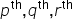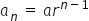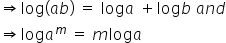Maths-
General
Easy

Question

# If l, m, n are theterms of a G.P which are +ve, then## 3    2    1    0Hint:

## The correct answer is: 0

### Given : l, m, n are theterms of a G.P  We know that in GPwhere, a is the first term r is the common ratio n is the number of the term which we want to find. Using this :Substituting these values in :We know thatUsing these formulas and further simplifyingThus,0#### With Turito Foundation.#### Get an Expert Advice From Turito.